Statistics (Mathematics) Class 9 - NCERT Questions
Q 1.

Give five examples of data that you can collect from your day-to-day life.

SOLUTION:

Following are five examples which are related to day-to-day life :
(i) Number of girl students in our class.
(ii) Number of computer sets in our computer lab.
(iii) Telephone bills of our house for last two years.
(iv) Number of students appeared in an examination obtained from newspapers.
(v) Number of female teachers in all the schools in a state obtained from the education department.

Q 2.

Classify the data in Q. 1 above as primary or secondary data.

SOLUTION:

We have:
Primary data: (i), (ii) and (iii)
Secondary data: (iv) and (v)

Q 3.

The blood groups of 30 students of Class VIII are recorded as follows:
A, B, O, O, AB, O, A, O, B, A, O, B, A, O, O,
A, AB, O, A, A, O, O, AB, B, A, O, B, A, B, O
Represent this data in the form of a frequency distribution table. Which is the most common, and which is the rarest, blood group among these students∴

SOLUTION: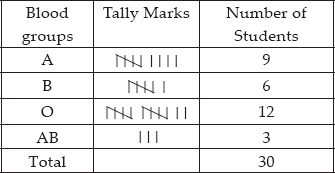From the above table, we have:
The most common blood group is O.
The rarest blood group is AB

Q 4.

The distance (in km) of 40 engineers from their residence to their place of work were found as follows:
5 3 10 20 25 11 13 7 12 31
19 10 12 17 18 11 32 17 16 2
7 9 7 8 3 5 12 15 18 3
12 14 2 9 6 15 15 7 6 12
Construct a grouped frequency distribution table with class size 5 for the data given above taking the first interval as 0-5 (5 not included). What main features do you observe from this tabular
representation∴

SOLUTION:

Here, the observation with minimum and maximum values are 2 and 32 respectively.
∴ The class intervals are as follows.
0 - 5, 5 - 10, 10 - 15, 15 - 20, 20 - 25, 25 - 30, 30 - 35
The required table is: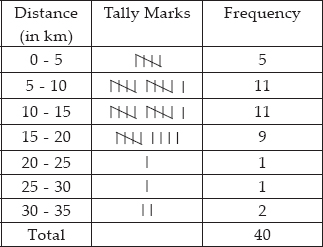From the above table we observe that:
(i) Frequencies of class interval 5 - 10 and 10 - 15 are equal, i.e. 11 each. It shows that maximum number of engineers have their residences at 5 to 15 km away from their work place.
(ii) Frequencies of class intervals 20 - 25 and 25 - 30 are also equal, i.e. 1 each. It shows that minimum number of engineers have their residences at 20 to 30 km away from their work place.

Q 5.

The relative humidity (in %) of a certain city for a month of 30 days was as follows:
98.1 98.6 99.2 90.3 86.5 95.3 92.9 96.3 94.2 95.1 89.2 92.3 97.1 93.5 92.7 95.1 97.2 93.3 95.2 97.3 96.2 92.1 84.9 90.2 95.7 98.3 97.3 96.1 92.1 89
(i) Construct a grouped frequency distribution table with classes 84 - 86, 86 - 88, etc.
(ii) Which month or season do you think this data is about∴
(iii) What is the range of this data∴

SOLUTION:

Here, the lowest value of observation = 84.9
The highest value of observation = 99.2
So, classes are : 84 - 86, 86 - 88, 88 - 90, ............, 98 - 100
(i) Thus, the required frequency table is: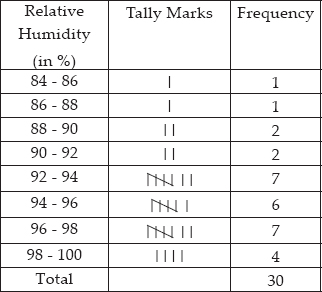(ii) Since, the relative humidity is high during the rainy season, so, the data appears to be taken in the rainy season.
(iii) Range = (Highest observation) - (Lowest observation) = 99.2 - 84.9 = 14.3

Q 6.

The heights of 50 students, measured to the nearest centimetres, have been found to be as follows:
161 150 154 165 168 161 154 162 150 151 162 164 171 165 158 154 156 172 160 170 153 159 161 170 162 165 166 168 165 164 154 152 153 156 158 162 160 161 173 166 161 159 162 167 168 159
158 153 154 159
(i) Represent the data given above by a grouped frequency distribution table, taking the class intervals as 160-165, 165-170, etc.
(ii) What can you conclude about their heights from the table∴

SOLUTION:

(i) Here, the lowest value of the observation = 150
The highest value of the observation = 173
∴ Classes are:
150 - 155, 155 - 160, ..., 170 - 175.
The frequency distribution table is :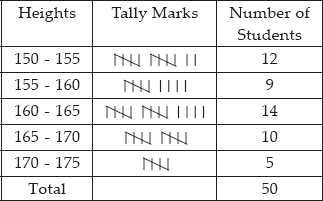(ii) More than 50% of the students are shorter than 165 cm.

Q 7.

A study was conducted to find out the concentration of sulphur dioxide in the air in parts per million (ppm) of a certain city. The data obtained for 30 days is as follows:
0.03 0.08 0.08 0.09 0.04
0.17 0.16 0.05 0.02 0.06
0.18 0.20 0.11 0.08 0.12
0.13 0.22 0.07 0.08 0.01
0.10 0.06 0.09 0.18 0.11
0.07 0.05 0.07 0.01 0.04

(i) Make a grouped frequency distribution table for this data with class intervals as 0.00-0.04, 0.04 -0.08, and so on.
(ii) For how many days, was the concentration of sulphur dioxide more than 0.11 parts per million∴

SOLUTION:

(i)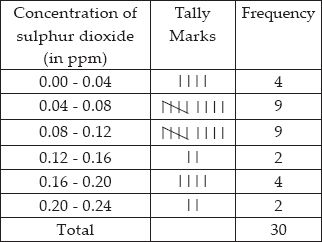(ii) The concentration of sulphur dioxide was more than 0.11 ppm for 8 days.

Q 8.

Three coins were tossed 30 times simultaneously. Each time the number of heads occurring was noted down as follows:
0 1 2 2 1 2 3 1 3 0
1 3 1 1 2 2 0 1 2 1
3 0 0 1 1 2 3 2 2 0
Prepare a frequency distribution table for the data given above.

SOLUTION: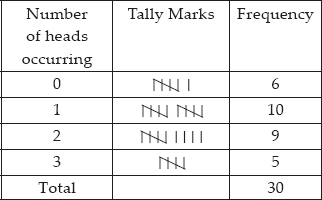Q 9.

The value of up to 50 decimal places is given below: 3.14159265358979323846264338327950288419716939937510
(i) Make a frequency distribution of the digits from 0 to 9 after the decimal point.
(ii) What are the most and the least frequently occurring digits∴

SOLUTION:

(i)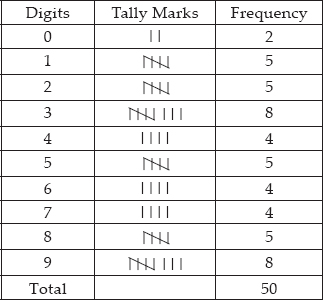(ii) The most frequently occurring digits are 3 and 9 and the least frequently occurring digit is 0.

Q 10.

Thirty children were asked about the number of hours they watched TV programmes in the previous week. The results were found as follows:
1 6 2 3 5 12 5 8 4 8
10 3 4 12 2 8 15 1 17 6
3 2 8 5 9 6 8 7 14 12
(i) Make a grouped frequency distribution table for this data, taking class width 5 and one of the class intervals as 5 -10.
(ii) How many children watched television for 15 or more hours a week∴

SOLUTION:

(i) The required frequency distribution is as follows.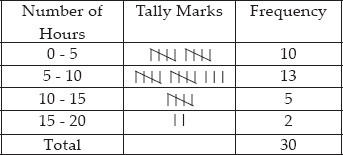(ii) Number of children who watched TV for 15 or more hours a week = 2.

Q 11.

A company manufactures car batteries of a particular type. The lives (in years) of 40 such batteries were recorded as follows:
2.6 3.0 3.7 3.2 2.2 4.1 3.5 4.5
3.5 2.3 3.2 3.4 3.8 3.2 4.6 3.7
2.5 4.4 3.4 3.3 2.9 3.0 4.3 2.8
3.5 3.2 3.9 3.2 3.2 3.1 3.7 3.4
4.6 3.8 3.2 2.6 3.5 4.2 2.9 3.6
Construct a grouped frequency distribution table for this data, using class intervals of size 0.5 starting from the interval 2-2.5.

SOLUTION: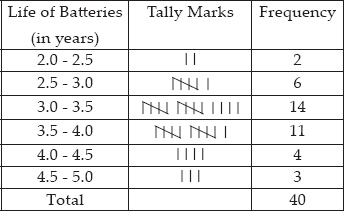Q 12.

A survey conducted by an organisation for the cause of illness and death among the women between the ages 15 - 44 (in years) worldwide, found the following figures (in %):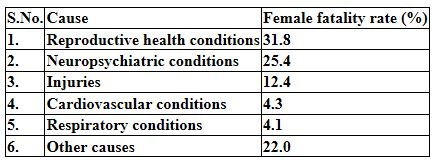(i) Represent the information given above graphically.
(ii) Which condition is the major cause of women's ill health and death worldwide∴
(iii) Try to find out, with the help of your teacher, any two factors which play a major role in the cause in (ii) above being the major cause.

SOLUTION:

(i) The required graphical representation is given below :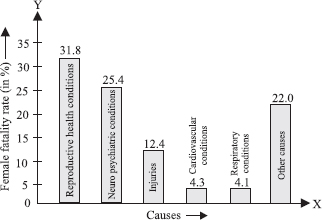(ii) The major cause of women's ill health worldwide' is 'reproductive health conditions'.
(iii) Two factors may be uneducation and poor background.

Q 13.

The following data on the number of girls (to the nearest ten) per thousand boys in different sections of Indian society is given below.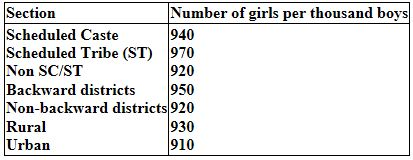(i) Represent the information above by a bar graph.
(ii) In the classroom discuss what conclusions can be arrived at from the graph.

SOLUTION:

(i) : The required bar graph is shown in the figure.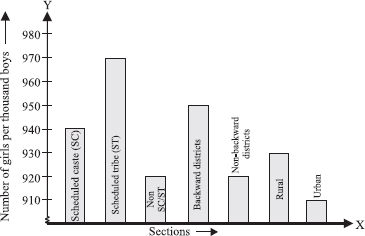(ii) Number of girls (per thousand boys) are maximum in scheduled tribes whereas minimum in urban.

Q 14.

Given below are the seats won by different political parties in the polling outcome of a state assembly elections: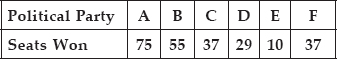(i) Draw a bar graph to represent the polling results.
(ii) Which political party won the maximum number of seats∴

SOLUTION:

(i) : The required bar graph is given below: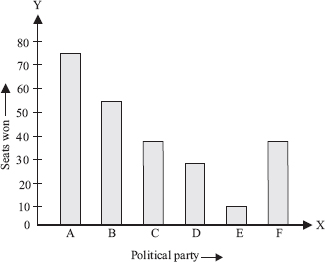(ii) The political party A won the maximum number of seats.

Q 15.

The length of 40 leaves of a plant are measured correct to one millimetre, and the obtained data is represented in the following table: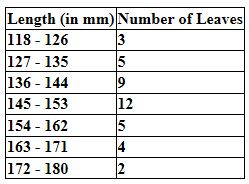(i) Draw a histogram to represent the given data.
(ii) Is there any other suitable graphical representation for the same data∴
(iii) Is it correct to conclude that the maximum number of leaves are 153 mm long∴ Why∴

SOLUTION:

(i) : The given frequency distribution is not continuous. Therefore, first we have to modify it to be continuous distribution.
Thus, the modified frequency distribution is: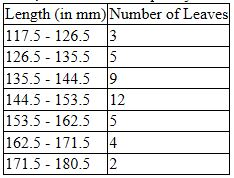Now, the required histogram of the above frequency distribution is as shown here: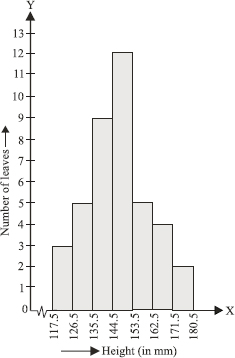(ii) Yes, other suitable graphical representation is a 'frequency polygon'.
(iii) No, it is not a correct statement. The maximum number of leaves are not 153 mm long rather they are from 145 mm to 153 mm long

Q 16.

The following table gives the life times of 400 neon lamps: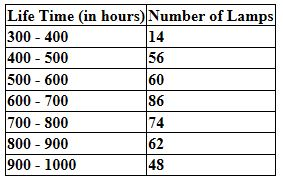(i) Represent the given information with the help of a histogram.
(ii) How many lamps have a life time of more than 700 hours.

SOLUTION:

(i) : The required histogram is shown as: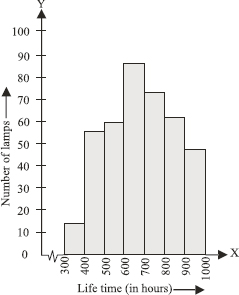(ii) Number of lamps having life time more than 700 hours = 74 + 62 + 48 = 184.

Q 17.

The following table gives the distribution of students of two sections according to the marks obtained by them.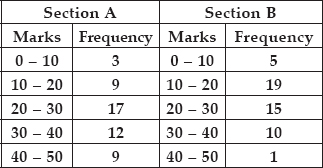Represent the marks of the students of both the sections on the same graph by two frequency polygons. From the two polygons compare the performance of the two sections.

SOLUTION:

To draw a frequency polygon we mark the class marks along x-axis. Therefore, the modified tables are: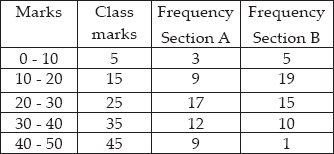So, the two frequency polygons are as shown below :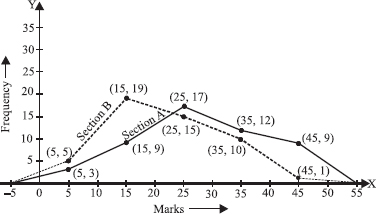More students of section A have secured good marks than students of section B.

Q 18.

The runs scored by two teams A and B on the first 60 balls in a cricket match are given below: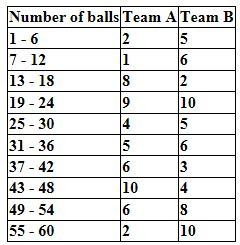Represent the data of both the teams on the same graph by frequency polygons.
[Hint: First make the class intervals continuous]

SOLUTION:

The given class intervals are not continuous. Therefore, we first modify the distribution as continuous.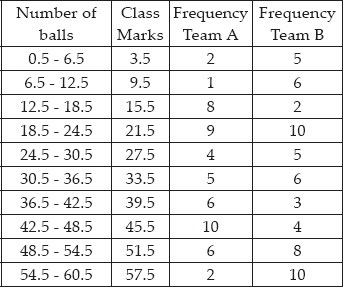Plotting the above ordered pairs on the same graph paper, we get: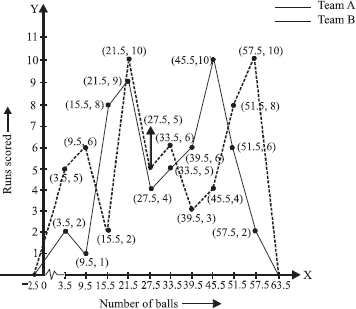Q 19.

A random survey of the number of children of various age groups playing in a park was found as follows :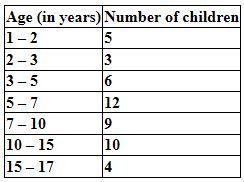Draw a histogram to represent the data above.

SOLUTION:

Here, the class sizes are different therefore, we calculate the adjusted frequencies corresponding to each rectangle. Adjusted frequency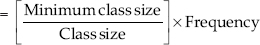Here, the minimum class size = 2 - 1 = 1
We have the following table of the adjusted frequencies: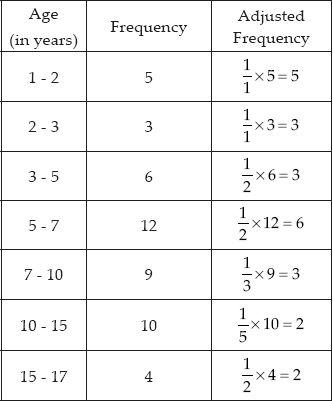Now, we draw the histogram taking Ages (in years) on the x-axis and corresponding adjusted frequencies on the y-axis as shown below: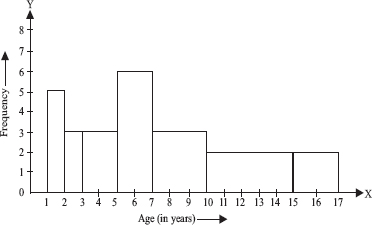Q 20.

100 surnames were randomly picked up from a local telephone directory and a frequency distribution of the number of letters in the English alphabet in the surnames was found as follows: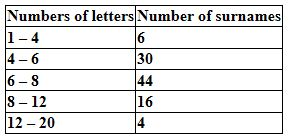(i) Draw a histogram to depict the given information.
(ii) Write the class interval in which the maximum number of surnames lie.

SOLUTION:

(i) Since, class intervals of the given frequency distribution are unequal, and the minimum class-size = 6 - 4 = 2. Therefore the adjusted frequencies are :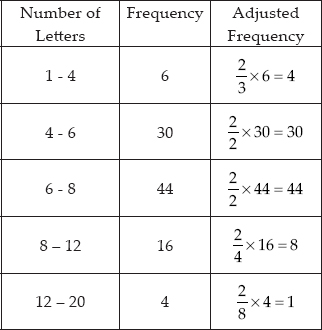The required histogram is given below: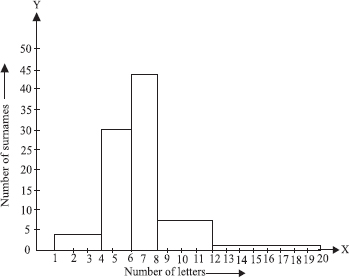(ii) The maximum frequency is 44, which is corresponding to the class interval 6 - 8.
∴ Maximum surnames lie in the class interval 6 - 8.

Q 21.

The following number of goals were scored by a team in a series of 10 matches:
2, 3, 4, 5, 0, 1, 3, 3, 4, 3
Find the mean, median and mode of these scores.

SOLUTION:

Here, n = 10.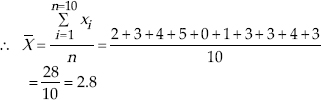∴ Mean = 2.8
Now arranging the given data in ascending order, we have: 0, 1, 2, 3, 3, 3, 3 4, 4, 5 For even observations,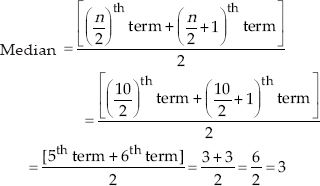Thus, the median of the data = 3
Forming frequency distribution table for the data, we get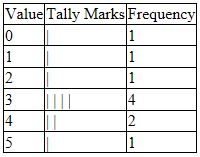So, Mode = 3

Q 22.

In a mathematics test given to 15 students, the following marks (out of 100) are recorded:
41, 39, 48, 52, 46, 62, 54, 40, 96, 52, 98, 40, 42, 52, 60
Find the mean, median and mode of this data.

SOLUTION:

To find the mean:
Here n = 15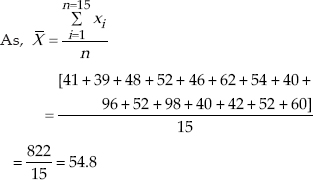Thus, mean = 54.8
To find median:
Arranging the given data in an ascending order, we have:
39, 40, 40, 41, 42, 46, 48, 52, 52, 52, 54, 60, 62, 96, 98
∴ n = 15, an odd number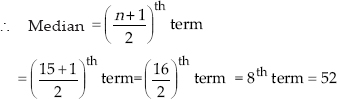Thus, median = 52
To find mode:
∴ In the given data, the observation 52 occurs 3 times, i.e. the maximum number of times.
∴ Mode = 52

Q 23.

The following observations have been arranged in ascending order. If the median of the data is 63, find the value of x.
29, 32, 48, 50, x, x + 2, 72, 78, 84, 95

SOLUTION:

Here, the given observations are in ascending order.
Since n = 10 (an even number of observations)
∴ Median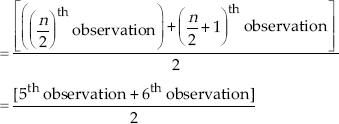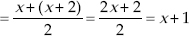Since, median = 63 [Given]
∴ x + 1 = 63 ∴ x = 63 - 1 = 62
Thus, the required value of x is 62.

Q 24.

Find the mode of 14, 25, 14, 28, 18, 17, 18, 14, 23, 22, 14, 18.

SOLUTION:

Arranging the given data in ascending order, we have 14, 14, 14, 14, 17, 18, 18, 18, 22, 23, 25, 28.
Since the observation 14 is occuring the maximum number of times (i.e. 4 times)
∴ Mode of the given data = 14

Q 25.

Find the mean salary of 60 workers of a factory from the following table: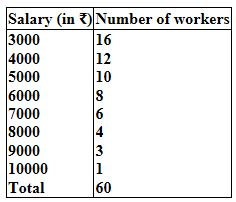SOLUTION: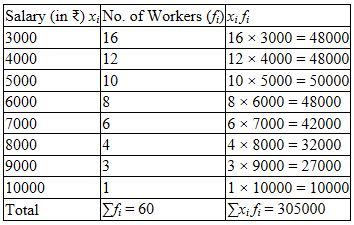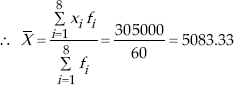Thus, the required mean salary = ₹ 5083.33

Q 26.

Give one example of a situation in which
(i) The mean is an appropriate measure of central tendency.
(ii) The mean is not an appropriate measure of central tendency but the median is an appropriate measure of central tendency.

SOLUTION:

(i) Mean height of the students of a class.
(ii) Median weight of a pen, a book, a rubber band a match box and a chair.